# How to show full column content in a PySpark Dataframe ?

• Last Updated : 06 Aug, 2021

Sometimes in Dataframe, when column data containing the long content or large sentence, then PySpark SQL shows the dataframe in compressed form means the first few words of the sentence are shown and others are followed by dots that refers that some more data is available.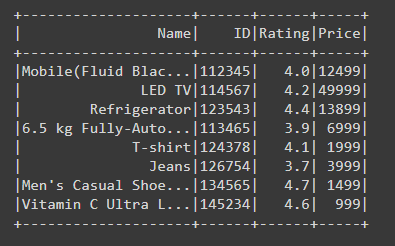From the above sample Dataframe, we can easily see that the content of the Name column is not fully shown. This thing is automatically done by the PySpark to show the dataframe systematically through this way dataframe doesn’t look messy, but in some cases, we are required to read or see the full content of the particular column.

So in this article, we are going to learn how to show the full column content in PySpark Dataframe. The only way to show the full column content we are using show() function.

Syntax: df.show(n, truncate=True)

Where df is the dataframe

• show(): Function is used to show the Dataframe.
• n: Number of rows to display.
• truncate: Through this parameter we can tell the Output sink to display the full column content by setting truncate option to false, by default this value is true.

Example 1: Showing full column content of PySpark Dataframe.

## Python

 `# importing necessary libraries``from` `pyspark.sql ``import` `SparkSession` `# function to create new SparkSession`  `def` `create_session():``    ``spk ``=` `SparkSession.builder \``        ``.master(``"local"``) \``        ``.appName(``"Product_details.com"``) \``        ``.getOrCreate()``    ``return` `spk`  `def` `create_df(spark, data, schema):``    ``df1 ``=` `spark.createDataFrame(data, schema)``    ``return` `df1`  `if` `__name__ ``=``=` `"__main__"``:` `    ``# calling function to create SparkSession``    ``spark ``=` `create_session()` `    ``input_data ``=` `[(``"Mobile(Fluid Black, 8GB RAM, 128GB Storage)"``,``                   ``112345``, ``4.0``, ``12499``),``                  ` `                  ``(``"LED TV"``, ``114567``, ``4.2``, ``49999``),``                  ` `                  ``(``"Refrigerator"``, ``123543``, ``4.4``, ``13899``),``                  ` `                  ``("``6.5` `kg Fully``-``Automatic Top Loading Washing Machine \``                  ``(WA65A4002VS``/``TL, Imperial Silver, Center Jet Technology)",``                   ``113465``, ``3.9``, ``6999``),``                  ` `                  ``(``"T-shirt"``, ``124378``, ``4.1``, ``1999``),``                  ` `                  ``(``"Jeans"``, ``126754``, ``3.7``, ``3999``),``                  ` `                  ``("Men's Casual Shoes ``in` `White Sneakers ``for` `Outdoor ``and``\``                  ``Daily use", ``134565``, ``4.7``, ``1499``),``                  ` `                  ``(``"Vitamin C Ultra Light Gel Oil-Free Moisturizer"``,``                   ``145234``, ``4.6``, ``999``),``                  ``]` `    ``schema ``=` `[``"Name"``, ``"ID"``, ``"Rating"``, ``"Price"``]``    ` `    ``# calling function to create dataframe``    ``df ``=` `create_df(spark, input_data, schema)` `    ``# visualizing full content of the Dataframe``    ``# by setting truncate to False``    ``df.show(truncate``=``False``)`

Output: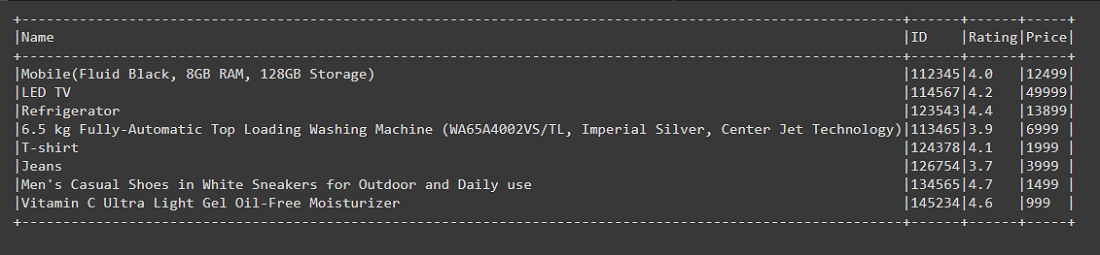Example 2: Showing Full column content of the Dataframe by setting truncate to 0.

In the example, we are setting the parameter truncate=0, here if we set any integer from 1 onwards such as 3, then it will show the column content up to three character or integer places, not more than that as shown in the below fig. But here in place of False if we pass 0 this will also act as the False, like in binary number 0 refers to false and show the full column content in the Dataframe.

## Python

 `# importing necessary libraries``from` `pyspark.sql ``import` `SparkSession` `# function to create new SparkSession``def` `create_session():``  ``spk ``=` `SparkSession.builder \``      ``.master(``"local"``) \``      ``.appName(``"Student_report.com"``) \``      ``.getOrCreate()``  ``return` `spk` `def` `create_df(spark,data,schema):``  ``df1 ``=` `spark.createDataFrame(data,schema)``  ``return` `df1` `if` `__name__ ``=``=` `"__main__"``:` `  ``# calling function to create SparkSession``  ``spark ``=` `create_session()``    ` `  ``input_data ``=` `[(``1``,``"Shivansh"``,``"Male"``,``80``,``"Good Performance"``),``          ``(``2``,``"Arpita"``,``"Female"``,``18``,"Have to work hard otherwise \``          ``result will ``not` `improve"),``          ``(``3``,``"Raj"``,``"Male"``,``21``,``"Work hard can do better"``),``          ``(``4``,``"Swati"``,``"Female"``,``69``,``"Good performance can do more better"``),``          ``(``5``,``"Arpit"``,``"Male"``,``20``,``"Focus on some subject to improve"``),``          ``(``6``,``"Swaroop"``,``"Male"``,``65``,``"Good performance"``),``          ``(``7``,``"Reshabh"``,``"Male"``,``70``,``"Good performance"``),``          ``(``8``,``"Dinesh"``,``"Male"``,``65``,``"Can do better"``),``          ``(``9``,``"Rohit"``,``"Male"``,``55``,``"Can do better"``),``          ``(``10``,``"Sanjana"``,``"Female"``,``67``,``"Have to work hard"``)]` `  ``schema ``=` `[``"ID"``,``"Name"``,``"Gender"``,``"Percentage"``,``"Remark"``]``  ` `  ``# calling function to create dataframe``  ``df ``=` `create_df(spark,input_data,schema)` `  ``# visualizing full column content of the dataframe by setting truncate to 0``  ``df.show(truncate``=``0``)`

Output: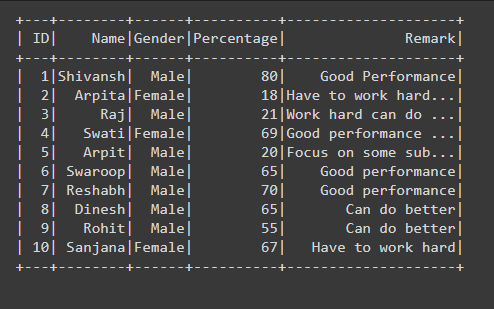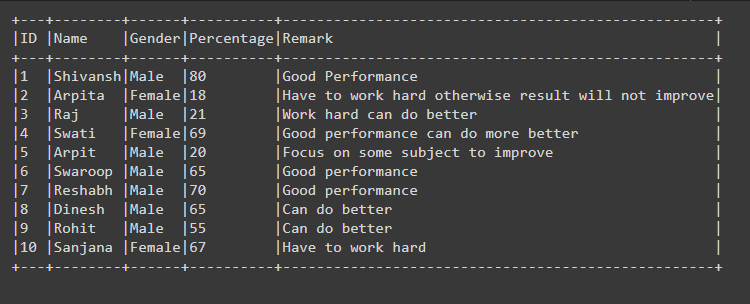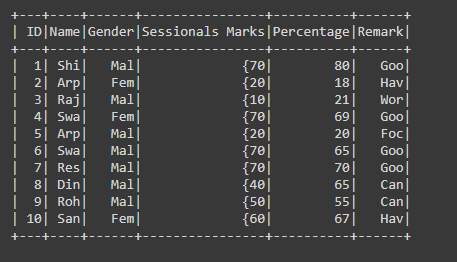Example 3: Showing Full column content of PySpark Dataframe using show() function.

In the code for showing the full column content we are using show() function by passing parameter df.count(),truncate=False, we can write as df.show(df.count(), truncate=False), here show function takes the first parameter as n i.e, the number of rows to show, since df.count() returns the count of the total number of rows present in the Dataframe, as in the above case total number of rows is 10, so in show() function n is passed as 10 which is nothing but the total number of rows to show.

## Python

 `# importing necessary libraries``from` `pyspark.sql ``import` `SparkSession` `# function to create new SparkSession`  `def` `create_session():``    ``spk ``=` `SparkSession.builder \``        ``.master(``"local"``) \``        ``.appName(``"Student_report.com"``) \``        ``.getOrCreate()``    ``return` `spk`  `def` `create_df(spark, data, schema):``    ``df1 ``=` `spark.createDataFrame(data, schema)``    ``return` `df1`  `if` `__name__ ``=``=` `"__main__"``:` `    ``# calling function to create SparkSession``    ``spark ``=` `create_session()` `    ``input_data ``=` `[(``1``, ``"Shivansh"``, ``"Male"``, (``70``, ``66``, ``78``, ``70``, ``71``, ``50``), ``80``,``                   ``"Good Performance"``),` `                  ``(``2``, ``"Arpita"``, ``"Female"``, (``20``, ``16``, ``8``, ``40``, ``11``, ``20``), ``18``,``                   ``"Have to work hard otherwise result will not improve"``),` `                  ``(``3``, ``"Raj"``, ``"Male"``, (``10``, ``26``, ``28``, ``10``, ``31``, ``20``),``                   ``21``, ``"Work hard can do better"``),``                  ` `                  ``(``4``, ``"Swati"``, ``"Female"``, (``70``, ``66``, ``78``, ``70``, ``71``, ``50``),``                   ``69``, ``"Good performance can do more better"``),``                  ` `                  ``(``5``, ``"Arpit"``, ``"Male"``, (``20``, ``46``, ``18``, ``20``, ``31``, ``10``),``                   ``20``, ``"Focus on some subject to improve"``),``                  ` `                  ``(``6``, ``"Swaroop"``, ``"Male"``, (``70``, ``66``, ``48``, ``30``, ``61``, ``50``),``                   ``65``, ``"Good performance"``),``                  ` `                  ``(``7``, ``"Reshabh"``, ``"Male"``, (``70``, ``66``, ``78``, ``70``, ``71``, ``50``),``                   ``70``, ``"Good performance"``),``                  ` `                  ``(``8``, ``"Dinesh"``, ``"Male"``, (``40``, ``66``, ``68``, ``70``, ``71``, ``50``),``                   ``65``, ``"Can do better"``),``                  ` `                  ``(``9``, ``"Rohit"``, ``"Male"``, (``50``, ``66``, ``58``, ``50``, ``51``, ``50``),``                   ``55``, ``"Can do better"``),``                  ` `                  ``(``10``, ``"Sanjana"``, ``"Female"``, (``60``, ``66``, ``68``, ``60``, ``61``, ``50``),``                   ``67``, ``"Have to work hard"``)]` `    ``schema ``=` `[``"ID"``, ``"Name"``, ``"Gender"``,``              ``"Sessionals Marks"``, ``"Percentage"``, ``"Remark"``]``    ` `    ``# calling function to create dataframe``    ``df ``=` `create_df(spark, input_data, schema)` `    ``# visualizing full column content of the``    ``# dataframe by setting n and truncate to``    ``# False``    ``df.show(df.count(), truncate``=``False``)`

Output: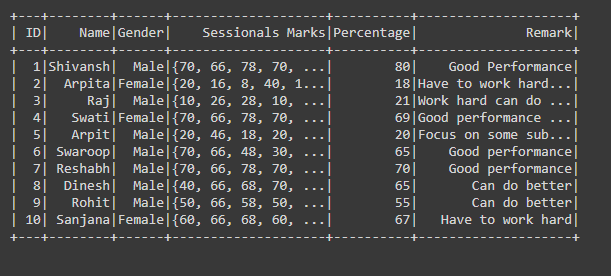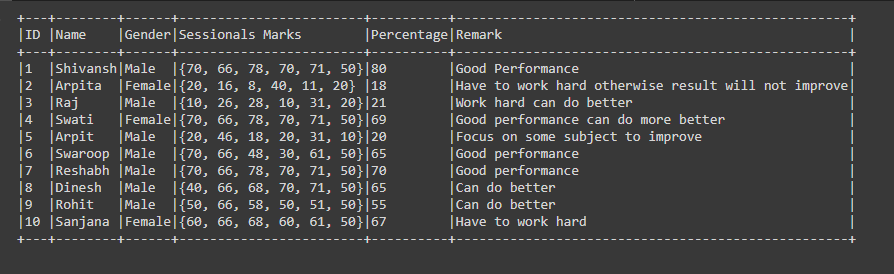My Personal Notes arrow_drop_up